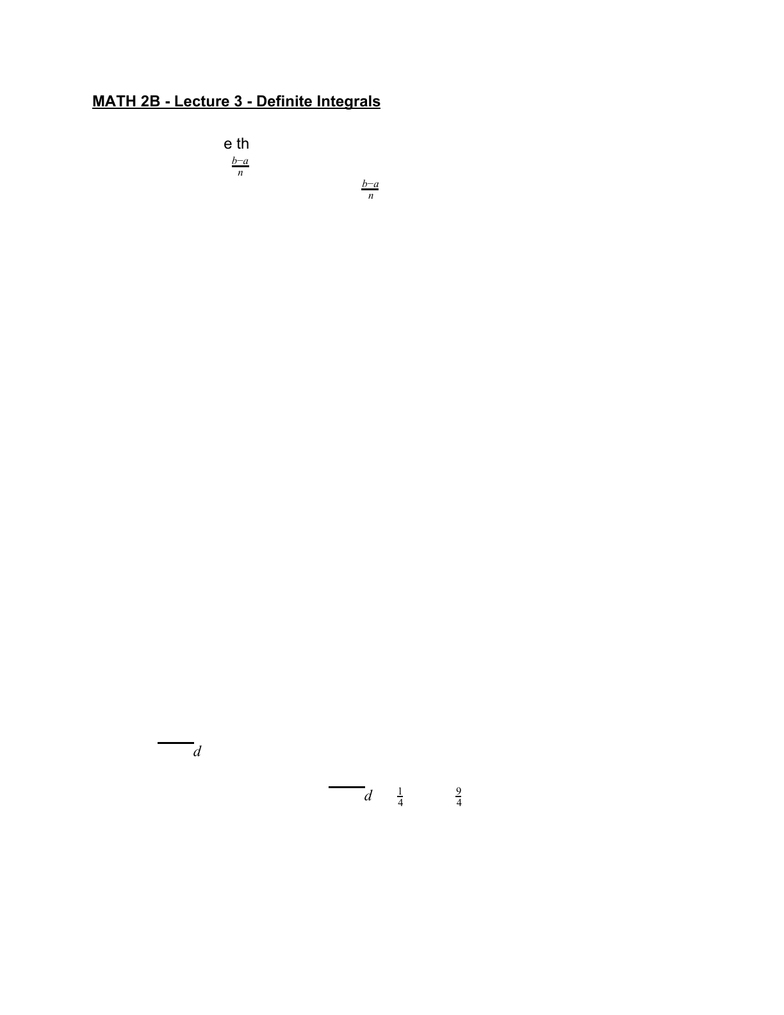Class Notes (1,100,000)
US (460,000)
UC-Irvine (10,000)
MATH (1,000)
MATH 2B (600)
Lecture 3

# MATH 2B Lecture 3: Definite IntegralsPremium

Department
Mathematics
Course Code
MATH 2B
Professor
ERJAEE, G.
Lecture
3

This preview shows half of the first page. to view the full 3 pages of the document.MATH 2B - Lecture 3 - Definite Integrals
Definition. Suppose that f is a function defined on an interval [a,b]. Let n be a positive
integer, define and letxΔ = n
ba
Δx i, for each i, , ..,xi=a+i=a+n
ba = 0 1 . n
Choose sample points . A Riemann Sum is any expression of the form ε [x, ]xi*i−1 xi
(xx
n
i=1
fi*
We say that the function is Riemann Integrable on [a,b] if converges tof(xxlim
n→∞
n
i=1
fi*
the same value for every choice of sample points. In such a case the definite integral of
f from a to b is:
(x)dx (xx
b
a
f= lim
n→∞
n
i=1
fi
Theorem
If f is continuous on [a,b], or has only a finite number of jump discontinuities, then
f is Riemann integrable on [a,b]
Net area under a curve
If , then = area under the curve (x)f≥ 0 (x)dx
b
a
f(x)y=f
If , then is negative
the area between the curve and the x-axis(x)f< 0 (x)dx
b
a
f< 0
In general difference between the areas above and below the x-axis(x)dx
b
a
f=
Example
1. represents the area of quarter circle of radius 3, hencedx
3
0
9 − x2
dx π π
3
0
9 − x2=4
1· 32=4
9
2. If f(x) = {x, x<2; 5-x, x 2 then the integral can be computed by≥ (x)dx
4
−1
f
summing/subtracting the areas shown below: